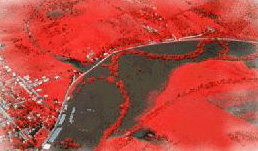Calculus on Demand at Dartmouth College Lecture 19 | Index | Lecture 21 Lecture 20## Resources

Math 3 Course Syllabus
Practice Exams

# Contents

In this lecture we learn how to sketch a curve (without graphing calculators) using the first and second derivative.

### Quick Question

Which are the graphs of a function, its first derivative, and its second derivative?### Textbook

Issues in Curve Sketching